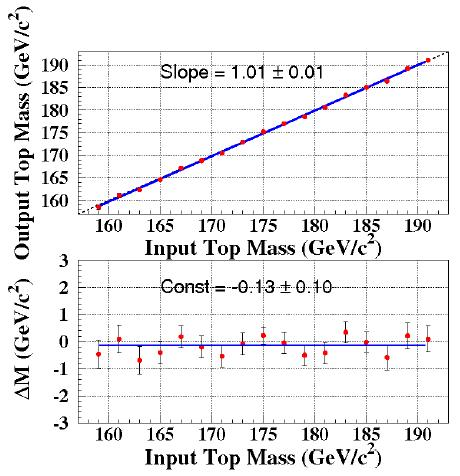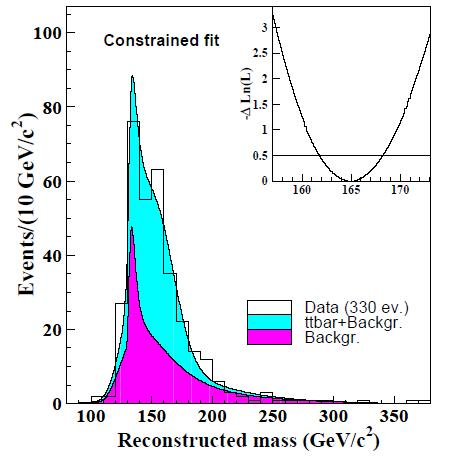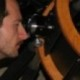## Top quark mass measured with neutrino phi weighting December 8, 2008

Posted by dorigo in news, physics, science.
Tags: , ,

I still remember when I moved the first steps in the world of experimental particle physics, a fresh new collaborator of the CDF experiment, in 1992. Back then, what the mass of the top quark could be was anybody’s guess. There were indications that the top was heavy, mainly due to the speed of oscillation of neutral B mesons; experimental searches had only set a limit which dictated that it had to be heavier than 91 GeV – about 97 protons. The top quark had to be heavy, but whether it was 120 or 150 or 200 GeV was really a favourite subject of speculations. We already knew, however, that a measurement would come first from a kinematic fit of single lepton decays.

Top quarks are mostly produced in pairs at hadron colliders. When they decay, top pairs always produce two b-quarks and two W bosons. While the b-quarks always end up fragmenting into a collimated stream of hadrons -what we call a jet-, W bosons may yield jets or lepton-neutrino pairs. Already in 1992, it was well-known that the single-lepton topology -the one arising when only one of the two W’s decayed into a lepton-neutrino pair, while the other yielded two additional hadronic jets- was the one which would discover the top, and which would allow its mass determination.

The single lepton topology includes a total of four jets, a lepton, and a neutrino. It is the best compromise between the number of signal events and the rate of mimicking background processes; but what’s more important, the escaping neutrino’s momentum can be figured out by the several constraints of the decay kinematics. You have two top quarks -their masses are the same-, then you have two W bosons -whose mass is 80.4 GeV-, and then you have overall a system whose transverse momentum components have to balance out. All in all, these are five constraints -five requirements on the observed momenta of the detected final state bodies- and since you only miss three components of the neutrino momentum, you can “solve the system” of equations, and determine both the neutrino momentum AND the top mass.

This is indeed how things went in 1994, when CDF published its first evidence for top quark production. Back then, the measurement of top quark mass using dilepton decays -ones arising when both W bosons decay into lepton-neutrino pairs- was considered unfeasible, or at least very unpractical.

In dilepton decays along with two b-quark jets and two charged leptons you get two neutrinos, and not just one: you thus have six unknown components of their momenta, and you need to know both if you want to reconstruct the decay kinematics completely. Because of those six unknowns, in the face of the five constraints listed above, the system is under-constrained: you cannot solve it, no more than you can give a univocal value to x,y,z in the system x+y+z=0,  x-y-z=1.

Brute-force computing comes to the rescue, however. The large statistics of dileptonic top pair decays collected by the CDF experiment in Run II -330 event candidates to play with- allows sophisticated statistical methods to be used, together with a good dose of number crunching. The idea is simple: even though the decay kinematics is unconstrained, one can make hypotheses for the neutrino momenta in the plane transverse to the beam direction (in this plane, a vector of missing energy does size up the combination of neutrino momenta), and to each hypothesis will correspond a reconstructed top quark mass, and an associated likelihood.

In fact, each of the objects detected in a dilepton top pair decay  -hadronic jets, charged leptons, missing transverse energy- is known within a well-determined uncertainty. Using the probability distribution function of each observable quantity, a likelihood can be computed. The method put together by CDF consists in scanning the angles of the two neutrinos in the transverse plane -their azimuthal angle phi– and determining the most likely top mass in each configuration. A simple weighting of the masses with the probability that neutrinos did in fact have those values of phi, given the measured momenta of jets and charged leptons, and the associated uncertainties, produces a very good estimate of the true top quark mass.

In the plot below you can see that the method allows to measure a top quark mass from a sample of dilepton decays which is almost exactly the same as the true one. For different samples of simulated top quark decays -each of them produced with a different top quark mass- the reconstructed mass matches perfectly the input one, and the output versus input points line up in a straight line bisecting the x,y axes.The second plot shows the difference between reconstructed and true mass as a function of the true (input) mass. The difference is consistent with zero, and there is no dependence of$\Delta M$ on the input mass: two features which make the measurement technique very solid and trustworthy.

In the end, one finds a “most likely” top quark mass per event, and a histogram of the latter can be drawn, and interpreted as the sum of background processes and signal. Monte Carlo simulations allow to predict the shape of backgrounds in this distribution, as well as the signal shape, once a particular top quark mass value is hypothesized. Different top quark masses produce different reconstructed mass distributions, such that from the distribution found in the data, the top mass can be derived.

Below you can see the mass distirbution obtained from 330 top pair candidates using the neutrino phi weighting technique. The data is represented by the black empty histogram; the fitted function consists of the sum of a background template (in magenta) and a signal template (in cyan). The inset shows the likelihood resulting from a fit of the distribution, as a function of the top quark mass. The minimum of the likelihood corresponds to the most likely top quark mass: 165.1 GeV.So, through a careful study of the mass distribution, CDF is able to measure a top mass of$165.1 \pm 3.3 \pm 3.1 GeV$ from 2.9 inverse femtobarns of data. This is a very precise result,  surpassing the combined precision that CDF and D0 had on the top mass at the end of Run I by using their single lepton decays. It shows that refined methods of analysis and measurement can overcome the difficulty of experimental situations. Neutrinos can be sized up despite the fact that we never measured one directly in our detector!

This new result by CDF does not alter much the world average value of the top quark mass; but it will almost certainly drive it down slightly, once it will be included in a global average. A lower top mass means a lower Higgs boson mass in global electroweak fits… And this makes things interesting for the searches of the Higgs boson, in two ways: first, because it increases the tension with current experimental upper limits (from LEP II:$M_H>114.4 GeV$), thus exposing the potential faults of the Standard Model; and second, because it makes it even more probable that the Higgs boson is in fact light -quite close to the 1.7-sigma excess found by LEP II in 2002. If the Higgs boson indeed weighs 115 GeV, it will take a while for LHC experiments to find it -this is the region of mass where less-than-straightforward decays have to be exploited to evidence a signal.

These are interesting times ahead of us! The options are still all on the table: no Higgs, a light Higgs, or a heavy one. Each of these has its own potential for a revolution of our understanding of the subnuclear world!

Back to the neutrino-phi weighting technique by CDF: I think it is worth mentioning, at the end of this post, that the improvements in the new analysis have produced a 20% better uncertainty -what’s equivalent to 44% more time spent running the experiment. As they say, a bit of analysis is worth a megabyte of code!1. Daniel de França MTd2 - December 9, 2008

What about the excess at 98GeV?2. dorigo - December 9, 2008

Hi Daniel,

which excess are you talking about ? Nothing I can see in the reconstructed to mass distribution. There, the data and the fit are in perfectly good agreement within uncertainties. But maybe I haven’t understood what you are pointing at.

Cheers,
T.3. Daniel de França MTd2 - December 9, 2008

Hi Tommaso!

This is something I found here:

On the 2nd paragraph of the introduction, p.2, it cites a mild excess at 98GeV.

Danieldorigo - December 9, 2008

Hi Daniel,

I see… Yes, there was this fluke at 98 GeV too. Nobody took it very seriously, although indeed you never know…

Cheers,
T.4. ali - December 9, 2008

hello,

just a dumb question,

why the 2nd plot is fit to a straight line while the data point show some kind of oscillation?!

ali5. Daniel de França MTd2 - December 9, 2008

Hi Tommaso!!

Frequently I see that you mention that the mass of the top is used to calculate the lower bound of the higgs mass. Can you sugest somewhere to find the calculation or formula, and the explanationg, relating both of them?

Daniel6. dorigo - December 15, 2008

Hi ali,

the residuals do appear to “oscillate” around the fit value, but there is no indication that that behavior is systematic, nor any reason why they should.

Hi Daniel,

sorry to not answer this – I overlooked your comment. I will send you a reference privately – it is in a slide of my subnuclear physics course.

Cheers,
T.7. dorigo - December 15, 2008

Hi ali,

the residuals do appear to “oscillate” around the fit value, but there is no indication that that behavior is systematic, nor any reason why they should.

Hi Daniel,

sorry to not answer this – I overlooked your comment. The delta rho parameter has a self-energy part which goes like$\Delta \rho = (3 G_F M_W^2)/(8 \sqrt 2 \pi^2) \times [m_t^2/m_W^2 - \tan^2 \theta_W (ln(m_H^2/m_W^2-5/6) + ... ]$

I will find a reference for you…

Cheers,
T.8. Daniel de França MTd2 - December 15, 2008

Tommaso,

Thanks, I found several things, but I don’t know what really I should trust. I need that because if the higgs turns so light, so close to the field instability level, that there might be something deeper to that. But I need a reference from someone that has a very experimental background because I want to think as clearly as possible.

Cheers,

Daniel. 🙂

Sorry comments are closed for this entry